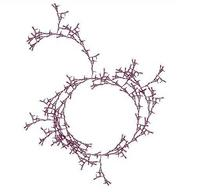University of Virginia, Department of Computer Science CS200: Computer Science, Spring 2003

Notes: Monday 31 March 2003

• Today, 5:00PM: Problem Set 8 team requests (see Notes 21 for details). The 5PM deadline is strict. We will assign the teams right after that.
• Friday, 4 April: Problem Set 7. Remaining lab hours: Tuesday, 1 April: 8-9:30pm (Jacques); Wednesday, 2 April: 8-9:30pm (Jacques)

Type Checking

What is a type?

What is the difference between manifest and latent types?

What is the difference between static and dynamic type checking?

How would Scheme be different if there was no type checking?

typeeval.ss

Code changes from meval.ss:
```;;; A very simple type system
;;;
;;; Type ::= PrimitiveType | ProcedureType | ProductType
;;; ProcedureType ::= Type -> Type
;;; ProductType ::= Type X Type
;;; PrimitiveType ::= Number | String | Boolean
;;;

(define (make-primitive-type type) (list 'primitive-type type))
(define (primitive-type? type) (tagged-list? type 'primitive-type))

(define (make-error-type) (list 'error-type))
(define (error-type? type) (tagged-list? type 'error-type))

(define (make-number-type) (make-primitive-type 'number))
(define (number-type? type) (and (primitive-type? type) (eq? (cadr type) 'number)))

(define (make-boolean-type) (make-primitive-type 'boolean))
(define (boolean-type? type) (and (primitive-type? type) (eq? (cadr type) 'boolean)))

(define (make-string-type) (make-primitive-type 'string))
(define (string-type? type) (and (primitive-type? type) (eq? (cadr type) 'string)))

(define (make-empty-type) (make-primitive-type 'empty))
(define (empty-type? type) (and (primitive-type? type) (eq? (cadr type) 'empty)))

(define (make-product-type type1 type2) (list 'product-type type1 type2))
(define (product-type? type) (tagged-list? type 'product-type))
(define (product-type-first type) (assert (product-type? type)) (caddr type))
(define (product-type-second type) (assert (product-type? type)) (cadr type))

(define (make-procedure-type params result) (list 'procedure-type params result))
(define (procedure-type? type) (tagged-list? type 'procedure-type))
(define (procedure-type-result type) (assert (procedure-type? type)) (caddr type))
(define (procedure-type-params type) (assert (procedure-type? type)) (cadr type))

(define (display-type type)
(cond ((procedure-type? type)
(string-append "(" (display-type (procedure-type-params type))
") -> (" (display-type (procedure-type-result type)) ")"))
((product-type? type)
(string-append (display-type (product-type-first type)) " x "
(display-type (product-type-second type))))
((number-type? type) "Number")
((boolean-type? type) "Boolean")
((string-type? type) "String")
((empty-type? type) "Void")
((error-type? type) "Error")
(else (error "Unknown type: " type))))

(define (parse-type type) ;;; Interprets a symbol or list of symbols as a type
(cond
((eq? type 'Number) (make-number-type))
((eq? type 'Boolean) (make-boolean-type))
((eq? type 'String) (make-string-type))
((list? type) (if (eq? (car type) '->)
(if (eq? (car type) 'X)
(else (error "Bad type form:" type))))

;;;
;;; We add types to our environment.  Every name now has both an associated
;;; type and an associated value.
;;;

(define (extend-environment names types values env)
(make-new-environment
(map (lambda (name type value) (list name type value)) names types values)
env))

(define (environment-lookup-value name env)
(if (null? env) (error "No binding for" name)
(if (frame-contains? name (first-frame env))
(frame-lookup-value name (first-frame env))
(environment-lookup-value name (enclosing-environment env)))))

(define (typeof-variable name env)
(if (null? env) (error "No binding for" name)
(if (frame-contains? name (first-frame env))
(frame-lookup-type name (first-frame env))
(typeof-variable name (enclosing-environment env)))))

(define (frame-lookup-value name frame)
(if (eq? (car (car frame)) name)
(frame-lookup-value name (cdr frame)))))

(define (frame-lookup-type name frame)
(if (eq? (car (car frame)) name)
(frame-lookup-type name (cdr frame)))))

;;;
;;; Defining typeof
;;;

(define (typeof expr env)
(cond
((self-evaluating? expr) (typeof-self-evaluating expr))
((variable? expr)        (typeof-variable expr env))
((lambda? expr)          (typeof-procedure expr env))
((application? expr)     (typeof-application expr env))
((definition? expr)      (typeof-definition expr env))
(else                    (error "Unknown expression: " exp))))

(define (typeof-self-evaluating expr)
(cond ((number? expr) (make-number-type))
((string? expr) (make-string-type))
((primitive-procedure? expr) (error "Can't tell type of primitive"))))

(define (typeof-sequence seq env)
(if (= (length seq) 1)
(typeof (car seq) env)
(begin
(typeof (car seq) env)
(typeof-sequence (cdr seq) env))))

(define (typeof-application expr env)
(let ((operator (typeof (application-operator expr) env)))
(if (procedure-type? operator)
(let ((argument-types (typelist-to-product-type
(map (lambda (operand) (typeof operand env))
(application-operands expr)))))
(if (type-match argument-types (procedure-type-params operator))
(procedure-type-result operator)
(begin
(printf "Type mismatch.~n  Application ~a parameter types are ~a, should be ~a."
expr (display-type argument-types)
(display-type (procedure-type-params operator)))
(make-error-type)))))))

(define (typeof-procedure expr env)
(let* ((params (lambda-parameters expr))
(body (lambda-body expr))
(param-types (map (lambda (param) (parse-type (cadr param))) params)))
(let ((restype (typeof-sequence
body
(extend-environment
(map (lambda (param) (car param)) params) ;; names
param-types
(map (lambda (param) 'unknown) params) ;; values
env))))
(make-procedure-type (typelist-to-product-type param-types) restype))))

(define (type-match t1 t2)
(cond
((or (error-type? t1) (error-type? t2)) #t) ;; error types match anything
((number-type? t1) (number-type? t2))
((boolean-type? t1) (boolean-type? t2))
((string-type? t1) (string-type? t2))
((procedure-type? t1)
(and (procedure-type? t2)
(type-match (procedure-type-params t1) (procedure-type-params t2))
(type-match (procedure-type-result t1) (procedure-type-result t2))))
((product-type? t1)
(and (product-type? t2)
(type-match (product-type-first t1) (product-type-first t2))
(type-match (product-type-second t1) (product-type-second t2))))
(else (error "Bad type: " t1))))

(define (parameter-types plist)
(typelist-to-product-type
(map (lambda (param) (parse-type (cadr param))) plist)))

(define (typelist-to-product-type typelist)
(if (null? typelist)
(make-empty-type)
(if (eq? (length typelist) 1)
(car typelist)
(make-product-type (car typelist)
(typelist-to-product-type (cdr typelist))))))

(define (check-procedure-definition expr env)
(let ((type (make-procedure-type
(parameter-types (lambda-parameters expr))
(parse-type (lambda-result-type expr))))
(params (lambda-parameters expr))
(body (lambda-body expr)))
(let ((restype
(typeof-sequence body
(extend-environment
(map (lambda (param) (car param)) params) ;; names
(map (lambda (param) (parse-type (cadr param))) params) ;; types
(map (lambda (param) 'unknown) params) ;; values
env))))
(if (type-match (procedure-type-result type) restype)
type
(begin
(printf
"Type mismatch. Procedure ~a evaluates to ~a, but declared to evaluate to ~a."
expr (display-type restype) (display-type (procedure-type-result type)))
(make-error-type))))))

(define the-global-environment
(make-new-environment
(list (list '+ (make-procedure-type
(make-product-type (make-number-type) (make-number-type))
(make-number-type))
(make-primitive-procedure +))
(list '* (make-procedure-type
(make-product-type (make-number-type) (make-number-type))
(make-number-type))
(make-primitive-procedure *)))
the-empty-environment))

(define (check-type expr) (display-type (typeof expr the-global-environment)))
```CS 200: Computer Science Department of Computer Science University of Virginia Circle Fractal by Ramsey Arnaoot and Qi Wang cs200-staff@cs.virginia.edu Using these Materials